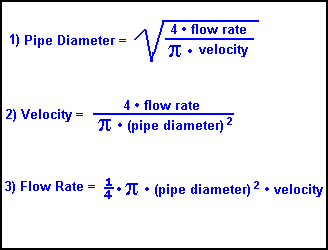# Need to find out the velocity of water

• Gregs6799
In summary, the task is to find the velocity in m/s of 3kg/s of fresh water traveling through a pipe with a diameter of 40 mm. The equation used is ##\Pi=S\cdot v##, where ##\Pi## is the volumetric flow rate, ##S## is the cross section area of the pipe, and ##v## is the velocity of the fluid. The solution involves converting the mass flow rate to volumetric flow rate and finding the cross section area of the pipe. The final answer is 2.3873 m/s.f

## Homework Statement

3kg/s of fresh water traveling through a pipe of 40 mm, I need to find out the velocity in m/s

## Homework Equations

I have tried the equation:
3 / 40

## The Attempt at a Solution

3 / 40 = = 0.075m/s
But not sure if I have to covert 3kg/s into another value before entering the equation but understand this is a weight value going into a speed value.
Any help very much appreciated.
Greg.

Last edited by a moderator:

## Homework Statement

3kg/s of fresh water traveling through a pipe of 40 mm, I need to find out the velocity in m/s

## Homework Equations

I have tried the equation:
3 / 40
That's not an equation -- it's just a number.
Gregs6799 said:

## The Attempt at a Solution

3 / 40 = = 0.075m/s
But not sure if I have to covert 3kg/s into another value before entering the equation but understand this is a weight value going into a speed value.
Any help very much appreciated.
Greg.
Start by finding the volume of 1 Kg of water, and the volume of the inside of the pipe. The inside volume of the pipe is its cross-section area time its length.

The equation is ##\Pi=S\cdot v##. Where ##\Pi## is the volumetric flow rate of fluid , ##S## is the cross section area of the pipe, and ##v ## is the velocity of the fluid.

We have ##\Pi## as ##kg/s## (we actually have the mass flow rate) but you first must convert it to ##m^3/s## (cubic meter per second). As Mark44 says, how much volume in cubic meters does 1kg of water occupy?

Then from the diameter (or is it radius) of 40mm you can calculate S but you got to find S with units of ##m^2## (square meter). And then you can find ##v## in the correct units (##m/s##).

Last edited: